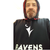# How to do data augmentation for deep learning model

281
10
4 weeks agoNew Contributor

Hi,

I got training data for my deep learning model by using export training data for deep learning tool in Arcgis Pro. I would like to do data augmentation on this training data. So, my code is

data=arcgis.learn.prepare_data(r'G:\data_training',
class_mapping={0: 'tree'}, chip_size=640, val_split_pct=0.1, batch_size=2,transforms=True,resize_to=800).

When I run the code, it has error:

```File C:\Program Files\ArcGIS\Pro\bin\Python\envs\arcgispro-py3\Lib\site-packages\arcgis\learn\_data.py, in prepare_data:
Line 1570:  data = (data.transform(transforms, **kwargs_transforms)

File C:\Program Files\ArcGIS\Pro\bin\Python\envs\arcgispro-py3\Lib\site-packages\fastai\data_block.py, in transform:
Line 504:   assert is_listy(tfms) and len(tfms) == 2, "Please pass a list of two lists of transforms (train and valid)."

AssertionError: Please pass a list of two lists of transforms (train and valid)```

Could you please suggest how I can fix this error? Thank you for your help

1 Solution

Accepted SolutionsOccasional Contributor II

Esri use a default set of transforms, but I have not been able to find them documented anywhere. Stated on

By default, prepare_data() uses a default set of transforms for data augmentation that work well for satellite imagery. These transforms randomly rotate, scale and flip the images so the model sees a different image each time. Alternatively, users can compose their own transforms using fast.ai transforms for the specific data augmentations they wish to perform.

You will have to dig into the code to find out what is being done for each model type. The prepare_data function is in C:\Program Files\ArcGIS\Pro\bin\Python\envs\arcgispro-py3\Lib\site-packages\arcgis\learn\_data.py

As an example, for MaskRCNN, this is in the code:

``````    if dataset_type == 'RCNN_Masks':
....
if transforms is None:
ranges = (0, 1)
if _image_space_used == _map_space:
train_tfms = [
crop(size=chip_size, p=1., row_pct=ranges, col_pct=ranges),
dihedral_affine(),
brightness(change=(0.4, 0.6)),
contrast(scale=(1.0, 1.5)),
rand_zoom(scale=(1.0, 1.2))
]
else:
train_tfms = [
crop(size=chip_size, p=1., row_pct=ranges, col_pct=ranges),
brightness(change=(0.4, 0.6)),
contrast(scale=(1.0, 1.5)),
rand_zoom(scale=(1.0, 1.2))
]
val_tfms = [crop(size=chip_size, p=1., row_pct=0.5, col_pct=0.5)]
tfms = (train_tfms, val_tfms)``````

So, it clear from the code that you need a list of training transforms and a list of validation transforms. Then they are combined into a tuple and that is what you put in the prepare_data transforms parameter.

Here is the documentation for the fastai transforms: https://fastai1.fast.ai/vision.transform.html

Something like this should get you started (the get_transforms function is an easy way, but you can individually create your own transforms also):

``````from fastai.vision.transform import get_transforms
tfms=get_transforms(do_flip=True, flip_vert=True, max_rotate=None, max_zoom=1, max_lighting=None, max_warp=None, p_affine=None, p_lighting=None, xtra_tfms=None)
tfms=(tfms1,tfms2)
data=arcgis.learn.prepare_data(r'G:\data_training', class_mapping={0: 'tree'}, chip_size=640, val_split_pct=0.1, batch_size=2,transforms=tfms,resize_to=800)``````

I haven't really played around with transforms much yet, so please let me know how you go.

10 RepliesbyMVP Notable Contributor

arcgis.learn module — arcgis 1.8.5 documentation

arcgis.learn.prepare_data(path, class_mapping=None, chip_size=224, val_split_pct=0.1, batch_size=64, transforms=None, collate_fn=<function _bb_pad_collate>, seed=42, dataset_type=None, resize_to=None, working_dir=None, **kwargs)

The first entry is supposed to be a data path, you passed it a string ( 'data_training' ).  If data_training is supposed to be the path, dump the single quotes

... sort of retired...New Contributor

My first entry is a path.byMVP Notable Contributor

see the single quotes around 'data_training'

that makes this a string

drop the single quotes like so..... data_training ...

If that is indeed the path

... sort of retired...New Contributor

my code :

data=arcgis.learn.prepare_data(r'G:\data_training',
class_mapping={0: 'tree'}, chip_size=640, val_split_pct=0.1, batch_size=2,transforms=True,resize_to=800)byMVP Notable Contributor

Ahhh I see from your revision history you fixed this arcgis.learn.prepare_data('data_training',

by adding the raw encoded G:\ to it.  Good.

At least that was ruled out

Next

Optional tuple. Fast.ai transforms for data augmentation of training and validation datasets respectively (We have set good defaults which work for satellite imagery well). If transforms is set to False no transformation will take place and chip_size parameter will also not take effect. If the dataset_type is ‘PointCloud’, use Transform3d class from arcgis.learn.

For transforms it says it is looking for a tuple.... you entered ... True

... sort of retired...New Contributor

Although I used the code  below, it still has the same error as in the originial post

data=arcgis.learn.prepare_data(r'G:\data_training',
class_mapping={0: 'tree'}, chip_size=640, val_split_pct=0.1, batch_size=2,transforms=True,resize_to=800)byMVP Notable Contributor

see my post... you missed the whole transforms thing

For transforms it says it is looking for a tuple.... you entered ... True

... sort of retired...New Contributor

tfms:Optional[Tuple[TfmList,TfmList]]. What should be TFmList? Is this the folders contain the train images and validation images?  How can I get TfmList?Occasional Contributor II

Esri use a default set of transforms, but I have not been able to find them documented anywhere. Stated on

By default, prepare_data() uses a default set of transforms for data augmentation that work well for satellite imagery. These transforms randomly rotate, scale and flip the images so the model sees a different image each time. Alternatively, users can compose their own transforms using fast.ai transforms for the specific data augmentations they wish to perform.

You will have to dig into the code to find out what is being done for each model type. The prepare_data function is in C:\Program Files\ArcGIS\Pro\bin\Python\envs\arcgispro-py3\Lib\site-packages\arcgis\learn\_data.py

As an example, for MaskRCNN, this is in the code:

``````    if dataset_type == 'RCNN_Masks':
....
if transforms is None:
ranges = (0, 1)
if _image_space_used == _map_space:
train_tfms = [
crop(size=chip_size, p=1., row_pct=ranges, col_pct=ranges),
dihedral_affine(),
brightness(change=(0.4, 0.6)),
contrast(scale=(1.0, 1.5)),
rand_zoom(scale=(1.0, 1.2))
]
else:
train_tfms = [
crop(size=chip_size, p=1., row_pct=ranges, col_pct=ranges),
brightness(change=(0.4, 0.6)),
contrast(scale=(1.0, 1.5)),
rand_zoom(scale=(1.0, 1.2))
]
val_tfms = [crop(size=chip_size, p=1., row_pct=0.5, col_pct=0.5)]
tfms = (train_tfms, val_tfms)``````

So, it clear from the code that you need a list of training transforms and a list of validation transforms. Then they are combined into a tuple and that is what you put in the prepare_data transforms parameter.

Here is the documentation for the fastai transforms: https://fastai1.fast.ai/vision.transform.html

Something like this should get you started (the get_transforms function is an easy way, but you can individually create your own transforms also):

``````from fastai.vision.transform import get_transforms
tfms=get_transforms(do_flip=True, flip_vert=True, max_rotate=None, max_zoom=1, max_lighting=None, max_warp=None, p_affine=None, p_lighting=None, xtra_tfms=None)
tfms=(tfms1,tfms2)
data=arcgis.learn.prepare_data(r'G:\data_training', class_mapping={0: 'tree'}, chip_size=640, val_split_pct=0.1, batch_size=2,transforms=tfms,resize_to=800)``````

I haven't really played around with transforms much yet, so please let me know how you go.